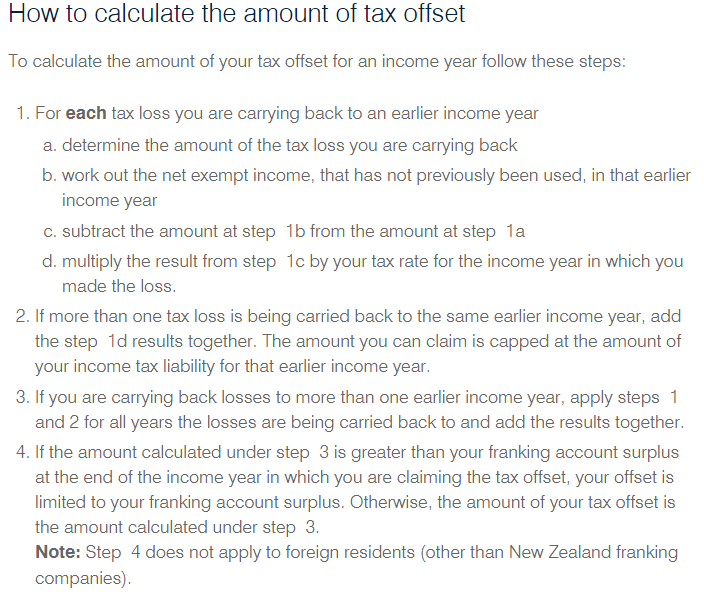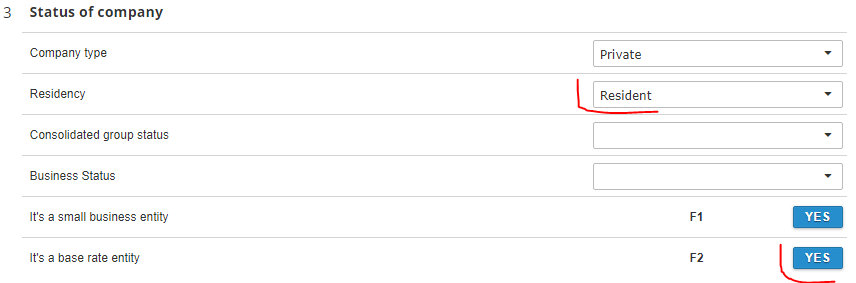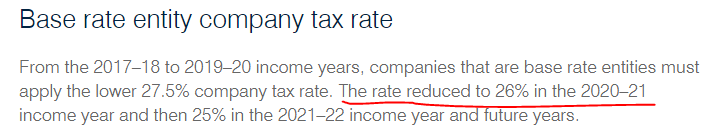"Loss carry back tax offset" calculation. Here is the article from ATO:According to it we're following instructions this way:

(1). A * G + (B - I) * rate + (C - J) * rate
(2). Then select minimum between (1) and L + M
(3). If company is AU resident, then select minimum between (2) and Franking balance

A, B, C, J, G, B, I , L, M - are labels on the Losses step (see screenshot below)
Franking balance is \$236.
As the company is a base rate entity then Rate is 26 %Losses schedule looks like this way:According to all above the calculations for these conditions will be:

(1). 167 * 0.275 + (732-0)*0.26 + (0-0)*0.26 = 45.925 + 189.54 = 236.25
(2). Then select minimum between (1) and 767 + 0 => 236.25
(3). If company is AU resident, then select minimum between (2) and 263 => 236

Thus, the Loss carry back tax offset will be \$236

Related guide from the ATO - https://www.ato.gov.au/Calculators-and-tools/Loss-carry-back-tax-offset-tool/

Note: Loss carry back tax offset cannot be more than Closing franking account balance. User can read how to complete franking account records here